## Calcudoku Puzzles

Calcudoku puzzles are a combination of arithmetic and logic type puzzles. Each calcudoku grid has blocks surrounded by bold lines. Each block has a math problem to solve based on arithmetic operations: addition, subtraction, multiplication or division. Numbers are placed in the blocks so that they occur only once in each row and once in each column. Similar to Sudoku. However, within each block, numbers can occur more than once. Remember: follow Sudoku puzzle rules for rows and columns.

Click for Larger Image andArithmetic Operations in Calcudoku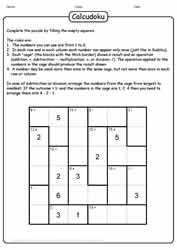Brain Health Supported by Calcudoku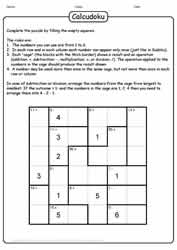Calcudoku Clue Supported Puzzle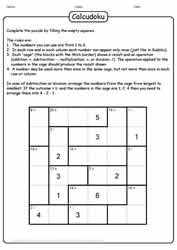Calcudoku Engages Reasoning SkillsCalcudoku Is Fun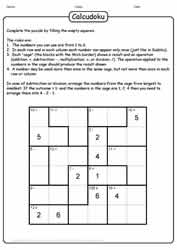Calcudoku Logic Puzzle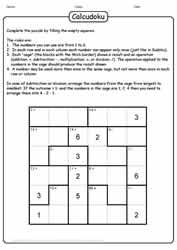Calcudoku Logics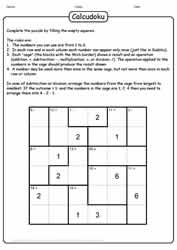Calcudoku Puzzle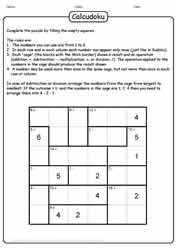Calcudoku Puzzle ChallengeCalcudoku Puzzle Like Sudoku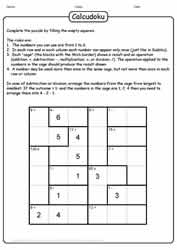Calcudoku Supports Brain Health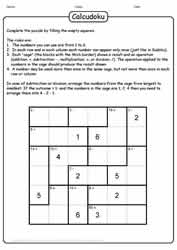Challenge the Brain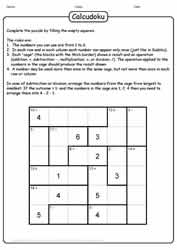Clues With Puzzle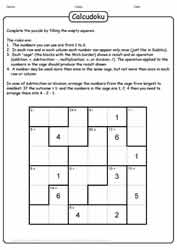Fun Calcudoku Puzzle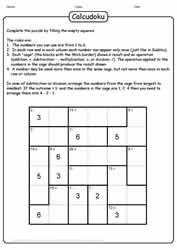Fun With Calcudoku PuzzleHint Supported Calcudoku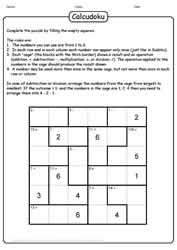Logic Calcudoku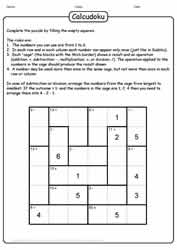Logic Calcudoku Puzzle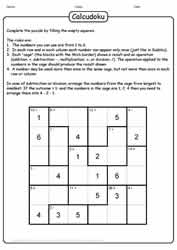Logic Puzzle CalcudokuLogic Puzzle Like Sudoku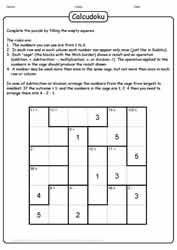Puzzle Calcudoku Challenge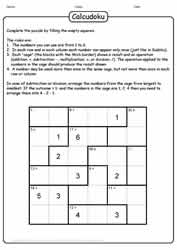Puzzle Supports Arithmetic Operations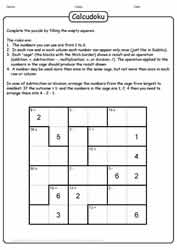Puzzle Supports Reasoning Skills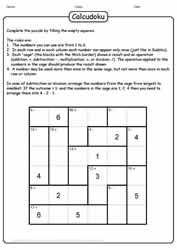Sudoku Like Calcudoku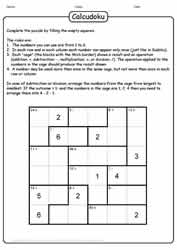Sudoku-Like Puzzle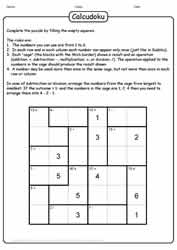#### SUMMER DAYS!

Here we are in the throes of summer, already! To help keep kids' brains active and healthy, here are direct links to a few of our summer activities puzzles collection. Word searches for kids | crossword puzzles for kids | word scramble.

Enjoy!